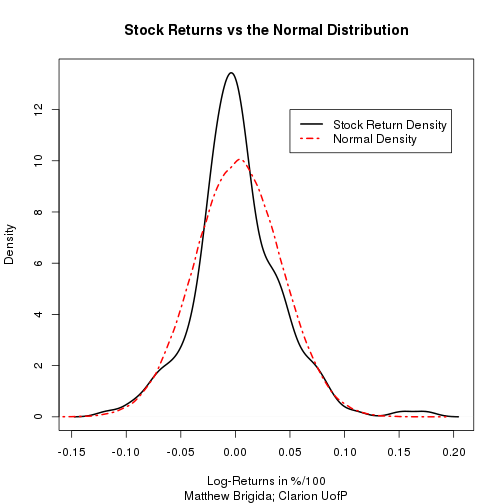# Skewness and Kurtosis R Code

Unfortulately all the R code on my blog didn't survive the conversion from blogger to wordpress. So I'll have to repost what I have saved, and write new code for the rest. Below is the code to test for skewness and kurtosis in a stocks returns.

The code also plots the empirical density function of the stocks returns and the normal density using the stock's mean return and standard deviation of returns. The idea of the plot is the empirical density describes how the stock behaved. The normal density is how you would have described the stock when using a model which assumes stock returns are normally distributed (assuming you also managed to predict the mean and standard deviation correctly). Any model based on greometric Brownian motion assumes returns are normal (such as Black-Scholes).

``````library(fBasics)
library(tseries)
``````
``````f <- function(x, y, l1, l2) {
## x is the stock ticker in quotes, y is the number of days of history you
## want, and l1 and l2 are the coordinates of the plot legend.

x <- get.hist.quote(x, start = (Sys.Date() - y), quote = "Close")
x <- ts(x)
r <- log(x[2:(length(x))]/x[1:(length(x) - 1)])

plot(density(r), main = "Stock Returns vs the Normal Distribution", lty = 1,
col = 1, lwd = 2, xlab = "Log-Returns in %/100", sub = "Matthew Brigida; Clarion UofP")

lines(density(rnorm(5e+05, mean = mean(r), sd = sd(r))), lty = 4, col = 2,
lwd = 2)

legend(l1, l2, c("Stock Return Density", "Normal Density"), col = c(1, 2),
lty = c(1, 4), lwd = c(2, 2))

cat("The sample skewness is", skewness(r), "\n")
cat("For a t-statistic of", skewness(r)/(sqrt(6/length(r))), "\n")
p1 <- 2 * (1 - pt(abs(skewness(r)/(sqrt(6/length(r)))), length(r) - 1))
cat("And a p-value of", p1, "\n")
cat("So we", ifelse(p1 < 0.05, "reject the null, and find the distribution is skewed.",
"do not reject the null, the distribution is symmetric."), "\n")
cat("\n")

cat("The sample excess kurtosis is", kurtosis(r), "\n")
cat("For a t-statistic of", kurtosis(r)/(sqrt(24/length(r))), "\n")
p2 <- 2 * (1 - pt(abs(kurtosis(r)/(sqrt(24/length(r)))), length(r) - 1))
cat("And a p-value of", p2, "\n")
cat("So we", ifelse(p2 < 0.05, "reject the null, and find the distribution has fat tails.",
"do not reject the null, the distribution does not have fat tails."),
"\n")
cat("\n")

rm(x)
rm(r)
rm(p1)
rm(p2)
}
``````

And we can use the function like:

``````f("cimt", 300, 0.05, 12)
``````
``````## time series starts 2013-04-01
````````````## The sample skewness is 0.5281
## For a t-statistic of 3.095
## And a p-value of 0.002246
## So we reject the null, and find the distribution is skewed.
##
## The sample excess kurtosis is 2.178
## For a t-statistic of 6.382
## And a p-value of 1.144e-09
## So we reject the null, and find the distribution has fat tails.
``````

So you can see form the output, we find evidence for both negative skewness and kurtosis in this stock's returns over the last 300 calendar days.